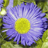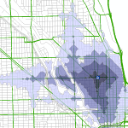# Join &quot;Lines&quot; result from OD Cost Matrix to point feature class for interpolation

2280
1
10-07-2013 06:48 AMNew Contributor
Hi,

My overall goal is to use network analyst (OD CostMatrix tool) to determine the haulcost (expressed as minutes) from each polygon (forest stand) to each mill in New Brunswick. I have the road network required and a points feature class designed to represent my polygon "origins" or starting points.

1) I need to create 77 cost matricies (one matrix for the travel time of each polygon in the province to 1 mill... there are 77 mills, so therefore 77 matricies to be created).

2)From there, I want to join each "lines" output from the OD CostMatrix to my point feature class (same as my "origins") and interpolate the travel time from each point to the travel time for each stand by creating a raster.

3)I will then use spatial zoning to apply the average raster value to my polygon (forest stand) layer.

I am automating the process since I need to complete the above steps for each of 77 mills. I am able to generate an OD cost matrix layer for each mill using python.

Here is my problem. I am unable to join "lines" to my point feature class; i.e. I cant' relate my cost matrix outputs back to the points of origin to create my raster layer.

How can I create a new feature class or select out my "lines" in the od cost matix layer so that I can join them to my point feature class?

I have tried arcpy.AddJoin_management - but it fails to exacute because my "join table" aka my "lines" output is not in an appropriate data format.

Can anyone help? I have attached the script I've created below... it works up until line 76 (where the OD cost matrix is solved). Some of the following commented out lines are my various attempts to turn my OD cost matrix outputs into something I can apply the AddJoin tool to. Advice would be much appreciated! Thanks!

- Heidi

# haulcosting.py
#
# Get haul time from each polygon to each mill in NB
#
#

import arcpy
import sys
from arcpy import env
import os

#
arcpy.CheckOutExtension("Network")
arcpy.CheckOutExtension ("spatial")

#Set environment
#

#Set variables for cost matrix
#
inNetworkDataset = "NBNET/NBNETWORK"
outNALayerName_base = "TimeTo"
impedenceAttribute = "Minutes"
accumulateAttributeName = ["Minutes","Meters"]
inOrigins = "ThirdJunctions"
inDestinations = "Mills/OperatingMills2012"
where_clause_base = '"DESTCODE" ='
OD_CostMatrix = "OD_CostMatrix"

print where_clause_base
print "So far good till looping"

#create new od cost matrix layer. Find minutes and meters to each mill
#
# Create List for Destinations
#
MillList = [row for row in arcpy.da.SearchCursor(inDestinations,["DESTCODE"])]

print "Creating Matrix layer"
outNALayer = arcpy.na.MakeODCostMatrixLayer(inNetworkDataset,"OD_CostMatrix","Minutes",accumulate_attribute_name = ["Minutes","Meters"],UTurn_policy = "NO_UTURNS",hierarchy= "USE_HIERARCHY",\
hierarchy_settings="",output_path_shape="STRAIGHT_LINES")
outNALayer = outNALayer.getOutput(0)
subLayerNames = arcpy.na.GetNAClassNames(outNALayer)

#Store sublayer names for origins and destinations
#
originsLayername = subLayerNames["Origins"]
destinationsLayerName = subLayerNames["Destinations"]

#Load ThirdJunctions as origins and map the DESTCODE field from mills feature as MillName
#
arcpy.na.AddLocations (outNALayer, originsLayername, inOrigins,"","#",snap_to_position_along_network = "SNAP")

# Generate Mill looping for Cost Matrix
#
for Mill in MillList:
print "Analyzing Mill" + Mill
outNALayerName = outNALayerName_base + Mill
outLayerFile = r"C:/Users/he1999/Desktop/FMP_Costs/Haul" + "/" + outNALayerName + ".lyr"
where_clause = where_clause_base + "'" + Mill + "'"
print where_clause
arcpy.MakeFeatureLayer_management(inDestinations,"temp",where_clause)
candiateFields = arcpy.ListFields("temp")
fieldmappings = arcpy.na.NAClassFieldMappings(outNALayer,destinationsLayerName,True,candiateFields)
arcpy.na.AddLocations (outNALayer, destinationsLayerName, "temp",fieldmappings,"#", append = "CLEAR")

#Solve OD cost matrix
#
print "Starting Solve"
arcpy.na.Solve (outNALayer, "SKIP")
print "Done"

#Save solved matrix layer as layer file on disk with relative paths
#
print "OD Saved as feature layer"

#arcpy.management.SaveToLayerFile(outNALayer,outLayerFile,"RELATIVE")
#print "Script completed successfully"

#print "Saved to Feature Class for" + Mill

#Join Lines from OD cost matrix to Third Junctions

layerName = inOrigins
joinTable = "OD_CostMatrix/Lines"
inField = "OBJECTID"
joinField = "OriginID"

#Select Data for join
arcpy.SelectData_management(outNALayer, "Lines")
print "Lines Selected"

print "Join Complete"

#Copy layer to a new permanent feature class"
arcpy.CopyFeatures_management(layerName, outFeature)
print "PointHaul join saved as feature for" + Mill

inPointFeatures = outFeature
zField = "Minutes"
cellSize = 100
power = 2

outIDW = Idw(inPointFeatures, zField, cellSize, power, searchRadius)

print "IDW Complete for" + Mill
break

arcpy.CheckInExtension("Network")
arcpy.CheckInExtension ("spatial")

print "We got er done Cowgirl!"
Tags (2)by`# ODLayer is the NA Layer object returned by getOutput(0)ODLayer = arcpy.MakeODCostMatrixLayer_na(inNetworkDataset, outNALayer_OD,                                impedanceAttribute, BufferSize, "",                                accumulate, uturns, restrictions,                                hierarchy, "", PathShape).getOutput(0)# To refer to the OD sublayers, get the sublayer names.if ArcVersion in ["10.1", "10.2"]:    naSubLayerNames = arcpy.na.GetNAClassNames(ODLayer)elif ArcVersion == "10.0":    naSubLayerNames = dict((sublayer.datasetName, sublayer.name) for sublayer in  arcpy.mapping.ListLayers(ODLayer)[1:])points = naSubLayerNames["Origins"]stops = naSubLayerNames["Destinations"]lines = naSubLayerNames["ODLines"]# Make layer objects for each sublayerlinesSubLayer = arcpy.mapping.ListLayers(ODLayer, lines)pointsSubLayer = arcpy.mapping.ListLayers(ODLayer, points)stopsSubLayer = arcpy.mapping.ListLayers(ODLayer, stops)# ... use sublayers in other tools`